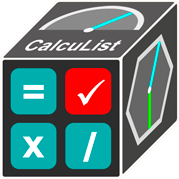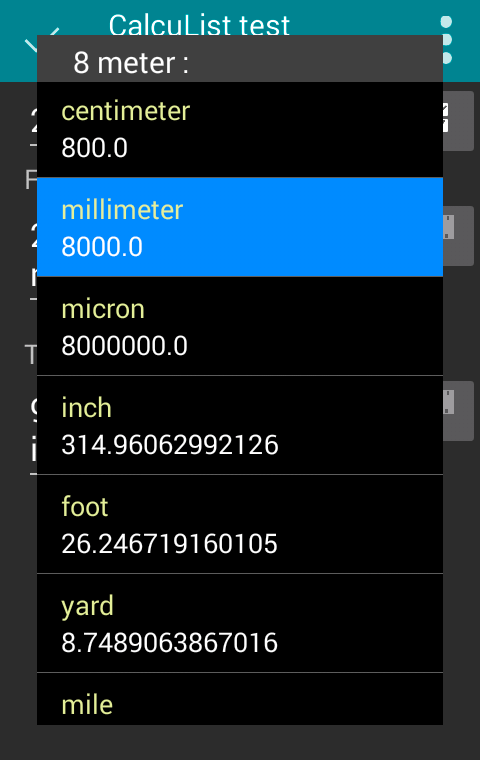CADinTools Loading

# CalcuList for Android

## developed by CADinTools## Create, delete and duplicate Calculation Lists#### Features to add to your lists:

1. Calculator
2. Unit Conversion: Length, angle, area, volumen and weight
3. Arc: Base-height, Base-length, Height-length, radius-length, Base-direction and radius-angle.
3. Triangle: Right Triangle and Triangle abc
5. Circle / Polygon
6. Notes

### CalculatorBasic calculations such as addition, subtraction, multiplication, division and Scientific operations such as trigonometric and logarithmic.

### Unit Conversione.g. Length conversion: 8 meters to millimeters

### Arce.g. Arc: base = 800 & height = 320

### Polygon / Circlee.g. Polygon: radius = 400 & 6 sides